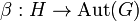# Trivial pair of actions is compatible

## Statement

Suppose$G$ and$H$ are groups and$\alpha:G \to \operatorname{Aut}(H)$ and$\beta:H \to \operatorname{Aut}(G)$ are group homomorphisms. Then, if$\alpha$ and$\beta$ are both trivial, they form a compatible pair of actions.

## Proof

We want to show that the trivial pair of actions is a compatible pair.

Want to show: If both the actions of the groups on each other are trivial, then the following holds, where$\cdot$ is interpreted from context as the action$\alpha$, the action$\beta$, or the action of a group on itself by conjugation:$g_1 \cdot (h \cdot g_2) = (g_1 \cdot h) \cdot (g_1 \cdot g_2) \ \forall \ g_1,g_2 \in G, h \in H$$h_1 \cdot (g \cdot h_2) = (h_1 \cdot g) \cdot (h_1 \cdot h_2) \ \forall g \in G, h_1,h_2 \in H$

Proof:

First equality, left side:$g_1 \cdot (h \cdot g_2) = g_1 \cdot g_2$ where we use the triviality of the action of$H$ on$G$.

First equality, right side:$(g_1 \cdot h) \cdot (g_1 \cdot g_2) = h \cdot (g_1 \cdot g_2) = g_1 \cdot g_2$ where the first step uses the triviality of the action of$G$ on$H$ and the second step uses the triviality of the action of$H$ on$G$.

Thus, the first equality holds.

Second equality, left side:$h_1 \cdot (g \cdot h_2) = h_1 \cdot h_2$ using the triviality of the action of$G$ on$H$.

Second equality, right side:$(h_1 \cdot g) \cdot (h_1 \cdot h_2) = g \cdot (h_1 \cdot h_2) = h_1 \cdot h_2$ where the first step uses the triviality of the action of$H$ on$G$ and the second step uses the triviality of the action of$G$ on$H$.